JEE  >  JEE Main Previous year questions (2016-22): The Solid State & Surface Chemistry

# JEE Main Previous year questions (2016-22): The Solid State & Surface Chemistry - Notes | Study Chemistry for JEE - JEE

 1 Crore+ students have signed up on EduRev. Have you?

Q.1. Atom X occupies the fcc lattice sites as well as alternate tetrahedral voids of the same lattice. The packing efficiency (in %) of the resultant solid is closest to      (JEE Advanced 2022)
(a)
25
(b)
35
(c)
55
(d)
75

Ans. b

Q.2. The correct option(s) related to adsorption processes is(are)      (JEE Advanced 2022)
(a) Chemisorption results in a unimolecular layer.
(b) The enthalpy change during physisorption is in the range of 100 to 140 kJ mol−1.
(c) Chemisorption is an endothermic process.
(d) Lowering the temperature favors physisorption processes.

Ans. a, d
(A) In Chemisorption unimolecular layer is formed.
(B) In Phesisorption 20-40 kJ of heat is released.
(C) Chemisorption is exothermic process.
(D) Physisorption increases with decrease in temperature.

Q.3. Given below are the critical temperatures of some of the gases: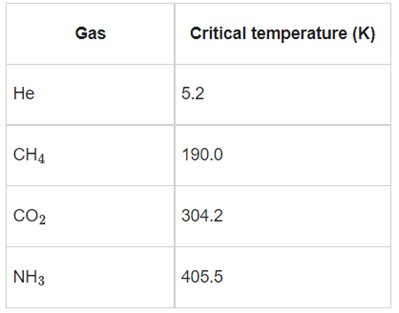The gas showing least adsorption on a definite amount of charcoal is           (JEE Main 2022)
(a) He
(b) CH4
(c) CO2
(d) NH3

Ans. a
The greater the value of critical temperature greater is adsorption as He has the least critical temperature so it adsorb least.

Q.4. Given below are two statements: One is labelled as Assertion A and the other is labelled as Reason R
Assertion A: Thin layer chromatography is an adsorption chromatography.
Reason R: A thin layer of silica gel is spread over a glass plate of suitable size in thin layer chromatography which acts as an adsorbent.
In the light of the above statements, choose the correct answer from the options given below:           (JEE Main 2022)

(a) Both A and R are true and R is the correct explanation of A
(b) Both A and R are true but R is NOT the correct explanation of A
(c) A is true but R is false
(d) A is false but R is true

Ans. a
Thin layer chromoatography (TLC) is another type of adsorption chromatography, which involve sepration of substance of a mixture ovel a thin layer of an adsorbent coated on glass plate.
A thin layer (about 0.2 mm thick) of an adsorbent (silica gel) or (Alumina) in spread overa glass plate of suitable size. Hence Assertion (A) is correct and Reason (R) is correct explanation of (A)

Q.5. An element X has a body centred cubic (bcc) structure with a cell edge of 200 pm. The density of the element is 5 g cm−3. The number of atoms present in 300 g of the element X is _______________.
Given: Avogadro constant, NA = 6.0 × 1023 mol−1.           (JEE Main 2022)
(a) 5 NA
(b) 6 NA
(c) 15 NA
(d) 25 NA

Ans. d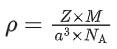Z = 2 for bcc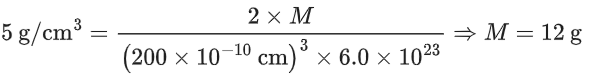12 g of element contain = NA atoms
300 g of element contains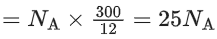Q.6. Which one of the following techniques is not used to spot components of a mixture separated on thin layer chromatographic plate?          (JEE Main 2022)
(a) I2 (Solid)
(b) U.V. Light
(c) Visualisation agent as a component of mobile phase
(d) Spraying of an appropriate reagent

Ans. c
TLC is a technique used to separate mixture of compounds based on differences in polarity. In TLC a glass plate coated with a stationary phase is spotted with the mixture to be separated.

Q.7. The incorrect statement about the imperfections in solids is:          (JEE Main 2022)
(a) Schottky defect decreases the density of the substance.
(b) Interstitial defect increases the density of the substance.
(c) Frenkel defect does not alter the density of the substance.
(d) Vacancy defect increases the density of the substance.

Ans. d
The vacancy defect increases the density of substance.
It does not change the density of the crystal. It only creates cationic vacancies. Frenkel Defect causes vacancy defect at its original site and an interstitial defect at its new location. Therefore, it does not change the density of the solid.

Q.8. Two statements are given below:
Statement I: The melting point of monocarboxylic acid with even number of carbon atoms is higher than that of with odd number of carbon atoms acid immediately below and above it in the series.
Statement II: The solubility of monocarboxylic acids in water decreases with increase in molar mass.

Choose the most appropriate option:          (JEE Main 2022)
(a) Both Statement I and Statement II are correct.
(b) Both Statement I and Statement II are incorrect.
(c) Statement I is correct but Statement II is incorrect.
(d) Statement I is incorrect but Statement II is correct.

Ans. a
Statement (I) is correct as monocarboxylic acids with even number of carbon atoms show better packing efficiency in the solid state, statement (II) is also correct as the solubility of carboxylic acids decreases with an increase in molar mass due to increase in the hydrophobic portion with an increase in the number of carbon atoms.

Q.9. Given below are two statements:
Statement I: Emulsions of oil in water are unstable and sometimes they separate into two layers on standing.
Statement II: For stabilisation of an emulsion, excess of electrolyte is added.
In the light of the above statements, choose the most appropriate answer from the options given below:          (JEE Main 2022)
(a) Both Statement I and Statement II are correct.
(b) Both Statement I and Statement II are incorrect.
(c) Statement I is correct but Statement II is incorrect.
(d) Statement I is incorrect but Statement II is correct.

Ans. c
Oil in water emulsions can sometimes separate into two layers on standing.
The most relevant example for the above case is milk, which can separate into two layers on standing for a longer time. Therefore, statement (I) is correct.
On adding excess of electrolyte, coagulation occurs and emulsion is further destabilised.
Therefore, statement (II) is incorrect.

Q.10. Ionic radii of cation A+ and anion B are 102 and 181pm respectively. These ions are allowed to crystallize into an ionic solid. This crystal has cubic close packing for BA+ is present in all octahedral voids. The edge length of the unit cell of the crystal AB is __________ pm. (Nearest Integer)          (JEE Main 2022)

Ans. 566
In cubic close packing, octahedral voids form at edge centers and body center of the cube
a = 2(rA+ + rB)
a = 2 (102 + 181)
a = 566 pm

Q.11. Metal M crystallizes into a fcc lattice with the edge length of 4.0 × 10−8 cm. The atomic mass of the metal is ____________ g/mol. (Nearest integer)
(Use: NA = 6.02 × 1023 mol−1, density of metal, M = 9.03 g cm−3 )         (JEE Main 2022)

Ans. 87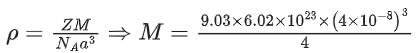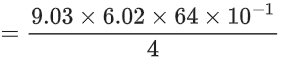= 86.9 g mol−1
≈ 87 g mol−1

Q.12. An element M crystallises in a body centred cubic unit cell with a cell edge of 300pmThe density of the element is 6.0 g cm−3. The number of atoms present in 180 g of the element is ____________ × 1023. (Nearest integer)          (JEE Main 2022)

Ans. 22
M is body certred cubic, ∴ Z = 2
Let mass of 1 atom of M is A
Edge length = 300pm
Density = 6 g/cm3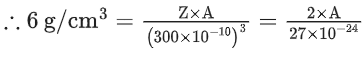A = 81 × 10−24 g
Atomic mass = 48.6 g
Mole in 180 g = 180 / 48.6 = 3.7 moles
Atoms of M = 3.7 × 6 × 1023 = 22.22 × 1023 atoms

Q.13. 100 mL of 0.3 M acetic acid is shaken with 0.8 g of wood charcoal. The final concentration of acetic acid in the solution after adsorption is 0.125 M. The mass of acetic acid adsorbed per gram of carbon is _____________ × 10−4 g.
(Given: Molar mass of acetic acid = 60 g mol
−1)          (JEE Main 2022)

Ans. 13125
Initial moles of acetic acid Initial moles of acetic acid = M× V1
= 100 × 0.3 = 30 m mol.
moles after adsorption = 100 × 0.125 = 12.5 m mol.
moles adsorbed = 30 − 12.5 = 17.5 m mol
moles = Weight / Molar Mass
weight = 17.5 × 10−3 × 60 =  1050 × 10−3 g
for 0.8 g charcoal mass of acetic acid adsorbed
= 1050 × 10−3 g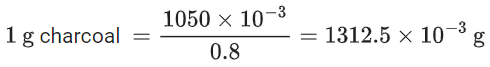= 13125 × 10−4 g

Q.14.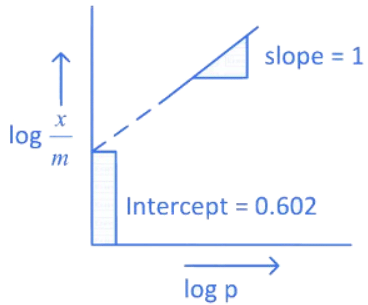If the initial pressure of a gas is 0.03 atm, the mass of the gas adsorbed per gram of the adsorbent is ___________ × 10−2 g.         (JEE Main 2022)

Ans. 12

Q.15. Metal deficiency defect is shown by Fe0.93O. In the crystal, some Fe2+ cations are missing and loss of positive charge is compensated by the presence of Fe3+ ions. The percentage of Fe2+ ions in the Fe0.93O crystals is __________. (Nearest integer)          (JEE Main 2022)

Ans. 85

Q.16. In a solid AB, A atoms are in ccp arrangement and B atoms occupy all the octahedral sites. If two atoms from the opposite faces are removed, then the resultant stoichiometry of the compound is AxBy. The value of x is ____________. [nearest integer]          (JEE Main 2022)

Ans. 3

Q.17. The distance between Na+ and Cl ions in solid NaCl of density 43.1 g cm−3 is _______________ × 10−10 m. (Nearest Integer)
(Given: NA = 6.02 × 1023 mol−1)
(JEE Main 2022)

Ans. 1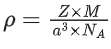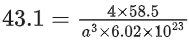a3 = 0.9 × 10−23
= 9 × 10−24
a = 2.08 × 10−8 cm
= 2.08 × 10−10 m
for NaCl, distance between Na+ and Cl− = a/2
= 1.04 × 10−10 m

Q.18. When 200 mL of 0.2 M acetic acid is shaken with 0.6 g of wood charcoal, the final concentration of acetic acid after adsorption is 0.1 M. The mass of acetic acid adsorbed per gram of carbon is ____________ g.           (JEE Main 2022)

Ans. 2
Mass of wood charcoal = 0.6 g
Initial moles of acetic acid = 0.2 × 0.2 = 0.04
Final moles of acetic acid = 0.1 × 0.2 = 0.02
Moles of acetic acid adsorbed = 0.04 − 0.02 = 0.02
Mass of acetic acid adsorbed per gm of charcoal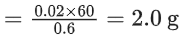Q.19. Atoms of element X form hcp lattice and those of element Y occupy 2/3 of its tetrahedral voids. The percentage of element X in the lattice is ____________. (Nearest integer)          (JEE Main 2022)

Ans. 43
Since X occupies hop lattice, Number of particles of type X in a unit cell = 6
Number of particles of type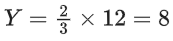∴ Percentage of element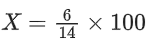/
= 300/7 = 42.85
≃ 43%

Q.20. For the given close packed structure of a salt made of cation X and anion Y shown below (ions of only one face are shown for clarity), the packing fraction is approximately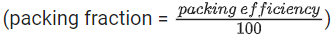(JEE Advanced 2021)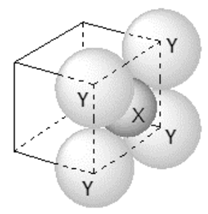(a) 0.74
(b) 0.63
(c) 0.52
(d) 0.48

Ans. b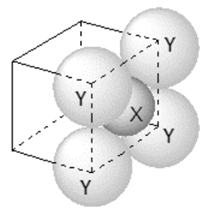Radius of cation X = r+
Number of cation X present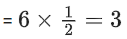Radius of anion Y = r-
Number of anion Y present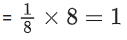Side of unit cell = a
As two anions are touching each other, so side of unit cell,
a = 2r
Volume of unit cell = a3 = (2r)3 = 8r
Volume of cations X = Number of cation × Volume of one cation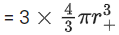Volume of anions Y = Number of anion × Volume of one anion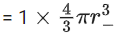Radius ratio for close packed structure,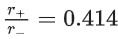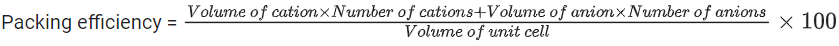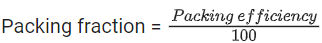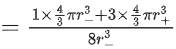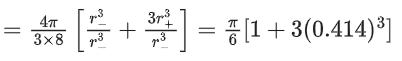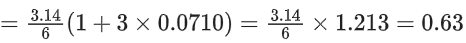Q.21. The correct statement(s) related to colloids is (are)                (JEE Advanced 2021)
(a) The process of precipitating colloidal sol by an electrolyte is called peptization.
(b) Colloidal solution freezes at higher temperature than the true solution at the same concentration.
(c) Surfactants form micelle above critical micelle concentration (CMC). CMC depends on temperature.
(d) Micelles are macromolecular colloids.

Ans. b, c
Statements (b) and (c) are correct whereas statements (a) and (d) are incorrect.
(a) Process of precipitating colloidal sol is known as coagulation.
(b) Concentration of colloidal solutions is small due to large molar mass and thus, their colligative properties are very small compared to true solutions. Hence, these solution freezes at higher temperature.
(c) Micelles are formed at the critical micelle concentration (CMC) which depends to temperature.
(d) Micelles and macromolecular colloids are two different types of colloid.

Q.22. The empirical formula for a compound with a cubic close packed arrangement of anions and with cations occupying all the octahedral sites in AxB. The value of x is _____________. (Integer answer)               (JEE Main 2021)

Ans. 1
Anions forms CCP or FCC(A) = 4A per unit cell Cations occupy all octahedral voids (B+) = 4B+ per unit cell
cell formula  A4B4
Empirical formula  AB  (x = 1)

Q.23. CH4 is absorbed on 1g charcoal at 0C following the Freundlich adsorpiton isotherm 10.0 mL of CH4 is adsorbed at 100 mm of Hg, whereas 15.0 mL is adsorbed at 200 mm of HgThe volume of CH4 adsorbed at 300 mm of Hg is 10x mL. The value of x is _________ × 10-2.
(Nearest integer) [Use log102 = 0.3010, log103 = 0.4771]               (JEE Main 2021)

Ans. 128
We know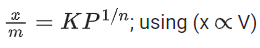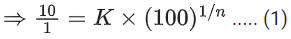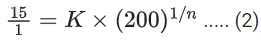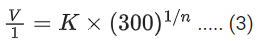Divide
(2) / (1)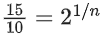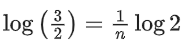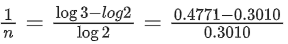1 / n = 0.585
Divide
(3) / (1)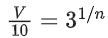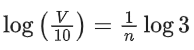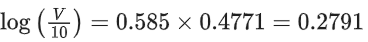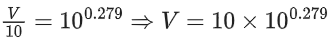⇒ V = 101.279 = 10x
x = 1.279
x = 128 × 10−2 (Nearest integer)

Q.24. CO2 gas adsorbs on charcoal following Freundlich adsorption isotherm. For a given amount of charcoal, the mass of CO2 adsorbed becomes 64 times when the pressure of CO2 is doubled. The value of n in the Freundlich isotherm equation is ____________ × 10−2(Round off to the Nearest Integer)               (JEE Main 2021)

Ans. 17
Freundlich isotherm;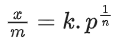Substituting values;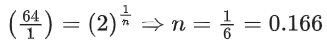≅ 17 × 10−2

Q.25. A copper complex crystallising in a CCP lattice with a cell edge of 0.4518 nm has been revealed by employing X-ray diffraction studies. The density of a copper complex is found to be 7.62 g cm−3. The molar mass of copper complex is ____________ g mol−1. (Nearest integer)
[Given: NA = 6.022 × 1023 mol−1]               (JEE Main 2021)

Ans. 106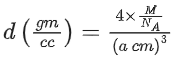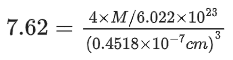⇒ M = 105.8 g/mol

Q.26. Diamond has a three dimensional structure of C atoms formed by covalent bonds. The structure of diamond has face centred cubic lattice where 50% of the tetrahedral voids are also occupied by carbon atoms. The number of carbon atoms present per unit cell of diamond is ____________.               (JEE Main 2021)

Ans. 8
Carbon atoms occupy FC lattice points as well as half of the tetrahedral voids.
Therefore number of carbon atoms per unit cell = 8

Q.27. 100 ml of 0.0018% (w/v) solution of Cl ion was the minimum concentration of Cl required to precipitate a negative sol in one h. The coagulating value of Cl ion is _________ (Nearest integer)               (JEE Main 2021)

Ans. 1
Coagulation value The minimum concentration of electrolyte in millimoles required to cause coagulation of 1 L of colloidal solution.
Given, 0.0018 g Cl present in 100 mL solution
Coagulation value of Cl is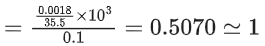Q.28. KBr is doped with 10−5 mole percent of SrBr2. The number of cationic vacancies in 1g of KBr crystal is ____________ 1014. (Round off to the Nearest Integer).
[Atomic Mass: K : 39.1 u, Br : 79.9 u NA = 6.023 × 1023]                (JEE Main 2021)

Ans. 5
For every Sr+2 ion, 1 cationic vacancy is created. Hence, no. of Sr+2 ion = Number of cationic vacancies
Since mole percentage of SrBr2 dropped is 10−5 to that of total moles of KBr.
Hence,
No. of cationic vacancy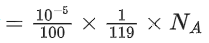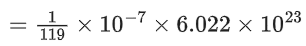= 5 × 10−2 × 10−7 × 1023 = 5 × 1014

Q.29. Ga (atomic mass 70 u) crystallizes in a hexagonal close packed structure. The total number of voids in 0.581 g of Ga is __________ × 1021. (Round off to the Nearest Integer).
[Given: NA = 6.023 × 1023]                (JEE Main 2021)

Ans. 15
For HCP structure Z = 6
Tetrahedral Void = (Z × 2) = 12
Octahedral Void = [Z × 1] = 6
No. of void per unit cell = 18
No. of unit cell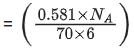No. of void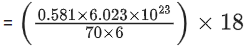= 14.99 × 1021

Q.30. A certain element crystallises in a bcc lattice of unit cell edge length 27oA. If the same element under the same conditions crystallises in the fcc lattice, the edge length of the unit cell in oA will be ____________. (Round off to the Nearest Integer).
[Assume each lattice point has a single atom]
[Assume 3 = 1.73, √2 = 1.41]                 (JEE Main 2021)

Ans. 33
For BCC unit cell, √3a = 4R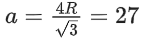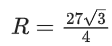For FCC unit cell
√2a = 4R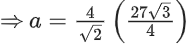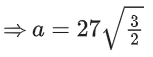⇒ a = 33.12 ≈ 33

Q.31. The number of octahedral voids per lattice site in a lattice is _________. (Rounded off to the nearest integer)                 (JEE Main 2021)

Ans. 1
Let us assume, the crystal has fcc or ccp lattice which has octahedral voids.
Number of lattice sites occupied = 8 corner + 6 face centres = 14.
Number of octahedral voids = 12 edge centres + 1 body centre = 13.
Number of octahedral void(s) per lattice site
= 13 / 14 = 0.928 ≃ 1

Q.32. The unit cell of copper corresponds to a face centered cube of edge length 3.596 oA with one copper atom at each lattice point. The calculated density of copper in kg/m3 is ___________. [Molar mass of Cu: 63.54 g; Avogadro Number = 6.022 × 1023]                 (JEE Main 2021)

Ans. 9077
Density of copper,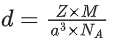Given, Z = 4, for fcc lattice,
M = 63.54 g mol−1
= 63.54 × 10−3 kg mol−1,
a = 3.596 Ao = 3.596 × 10−10 m,
NA = 6.022 × 1023 mol−1
On putting given values, we get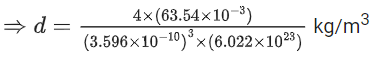= 9076.26 ≃ 9077 kg/m3

Q.33. The coordination number of an atom in a body-centered cubic structure is _______.
[Assume that the lattice is made up of atoms.]                 (JEE Main 2021)

Ans. 8
Coordination number is the number of nearest neighbours of a central atom in the structure.
bcc has a coordination number of 8 and contains 2 atoms per unit cell.
This is because each atom touches four atoms in the layer above it, four in the layer below it and none in its own layer.

Q.34. Select the graph that correctly describes the adsorption isotherms at two temperatures T1, and T2 (T1 > T2) for a gas:
(x - mass of the gas absorbed ; m - mass of adsorbent ; P - pressure)                  (JEE Main 2021)
(a)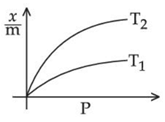(b)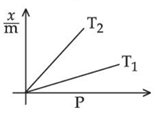(c)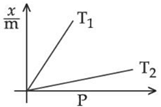(d)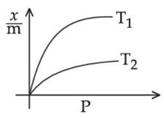Ans. a
According to Freundlich adsorption isotherm, in the median range of pressure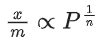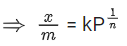On increasing temperature x/m decreases.
Adsorption is generally exothermic in nature. Hence, correct option is (A).

Q.35. Given below are two statements.
Statement I: Frenkel defects are vacancy as well as interstitial defects.
Statement II: Frenkel defect leads to colour in ionic solids due to presence of F-centres.
Choose the most appropriate answer for the statements from the options given below:                  (JEE Main 2021)
(a) Statement I is false but Statement II is true
(b) Both Statement I and Statement II are true
(c) Statement I is true but Statement II is false
(d) Both Statement I and Statement II are false

Ans. c
Frenkel defect is vacancy as well as interstitial defect as atom leaves its place in lattice creating a vacancy and occupy interstitial site in a nearby location. Hence, statement I is true.
Frenkel defect does not leads to colour in ionic solids due to absence of F-centres. Ionic solids having anionic vacancies because of metal excess defects. This leads to colour in ionic solids due to presence of F-centres. Hence, statement II is false.

Q.36. Which one of the following is correct for the adsorption of a gas at a given temperature on a solid surface?                  (JEE Main 2021)
(a) ΔH > 0, ΔS > 0
(b) ΔH > 0, ΔS < 0
(c) ΔH < 0, ΔS < 0
(d) ΔH < 0, ΔS > 0

Ans. c
(i) Adsorption of gas at metal surface is an exothermic process of ΔH < 0
(ii) As the adsorption of gas on metal surface reduces the free movement of gas molecules thus restricting its randomness hence ΔS < 0

Q.37. Select the correct statements:
(A) Crystalline solids have long range order.
(B) Crystalline solids are isotropic.
(C) Amorphous solid are sometimes called pseudo solids.
(D) Amorphous solids soften over a range of temperatures.
(E) Amorphous solids have a definite heat of fusion.
Choose the most appropriate answer from the options given below:                   (JEE Main 2021)
(a) (A), (B), (E) only
(b) (B), (D) only
(c) (C), (D) only
(d) (A), (C), (D) only

Ans. d
(A) Crystalline solids have definite arrangement of constituent particles and have long range order.
(C), (D) Different constituent particles of an amorphous solid have different bond strengths and soften over a range of temperatures.

Q.38. The parameters of the unit cell of a substance are a = 2.5, b = 3.0, c = 4.0, α = 90, β = 120, γ = 90. The crystal system of the substance is:                   (JEE Main 2021)
(a) Hexagonal
(b) Orthorhombic
(c) Monoclinic
(d) Triclinic

Ans. c
b  c and α = γ = 90  β are parameters of monoclinic unit cell.

Q.39. A hard substance melts at high temperature and is an insulator in both solid and in molten state. This solid is most likely to be a/an:                   (JEE Main 2021)
(a) Covalent solid
(b) Molecular solid
(c) Ionic solid
(d) Metallic solid

Ans. a
Covalent or network solid are insulator (except graphite) and have very high melting point.

Q.40. In a binary compound, atoms of element A form a hcp structure and those of element M occupy 2/3 of the tetrahedral voids of the hcp structure. The formula of the binary compound is:                   (JEE Main 2021)
(a) M2A3
(b) M4A3
(c) M4A
(d) MA3

Ans. b
In HCP unit cell,
Z = 6 so A = 6
Also we know, in HCP
Tetrahedral voids = 2Z = 12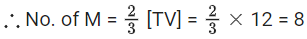Formula = M8A6 = M4A3

Q.41.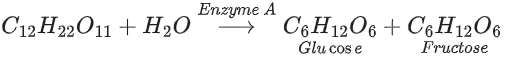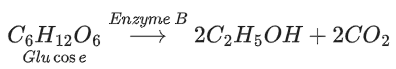In the above reactions, the enzyme A and enzyme B respectively are:                   (JEE Main 2021)
(a) Zymase and Invertase
(b) Invertase and Amylase
(c) Amylase and Invertase
(d) Invertase and Zymase

Ans. d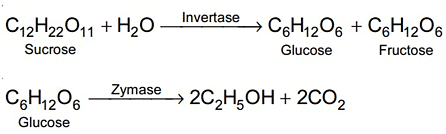Enzyme A is Invertase
Enzyme B is Zymase

Q.42. The nature of charge on resulting colloidal particles when FeCl3 is added to excess of hot water is:                    (JEE Main 2021)
(a) neutral
(b) sometimes positive and sometimes negative
(c) positive
(d) negative

Ans. c
If FeCl3 is added to excess of hot water, a positively charged sol of hydrated ferric oxide is formed due to adsorption of Fe3+ ions.

Q.43. Which one of the following statements is FALSE for hydrophilic sols?                    (JEE Main 2021)
(a) They do not require electrolytes for stability.
(b) The sols cannot be easily coagulated.
(c) These sols are reversible in nature.
(d) Their viscosity is of the order of that of H2O.

Ans. d
1. Viscosity of hydrophilic sol > viscosity of H2O.
2. Hydrophilic sol is more stable so can't be easily coagulated.
3. Hydrophilic sols are reversible sols.
4. No electrolytes are required to stabilise hydrophilic sol.

Q.44. In Freundlich adsorption isotherm at moderate pressure, the extent of adsorption (x/m) is directly proportional to Px. The value of x is:                     (JEE Main 2021)
(a) ∞
(b) zero
(c) 1
(d) 1/n

Ans. d
According to Freundlich adsorption isotherm, in the median range of pressure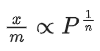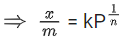Hence, x = 1/n

Q.45. In Freundlich adsorption isotherm, slope of AB line is:                    (JEE Main 2021)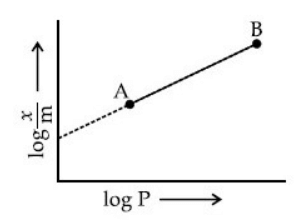(a)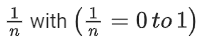(b) n with (n, 0.1 to 0.5)
(c)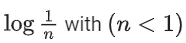(d) log n with (n > 1)

Ans. a
According to Freundlich adsorption isotherm, in the median range of pressure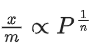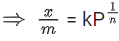taking log both sides, we get,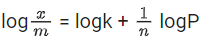For straight line, comparing above equation to y = mx + c
So, m = slope = 1/n
Hence 0  1/n  1
It value lies in between 0 to 1.

Q.46. Which of the following compounds is likely to show both Frenkel and Schottky defects in its crystalline form?    (2020)
(a) AgBr
(b) CsCl
(c) KBr
(d) ZnS

Ans. a
In AgBr, there in not very large difference between the size of the cation and anion, thus, it will show both Frenkel and Schottky defect in crystalline form.

Q.47. At 100°C, copper (Cu) has FCC unit cell structure with cell edge length of x Å. What is the approximate density of Cu (in g cm-3) at this temperature?
[Atomic Mass of Cu = 63.55 u]    (2019)
(a) 205/x3
(b) 105/x3
(c) 211/x3
(d) 422/x3

Ans. d
We know that,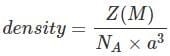Given that: Z = 4(fcc)
M= 63.5 g
NA = 6 × 1023
a = x × 10-8cm
After putting the values, we get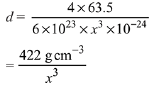Q.48. Which primitive unit cell has unequal edge lengths (a ≠ b ≠ c) and all axial angles different from 90°?    (2019)
(a) Triclinic
(b) Hexagonal
(c) Monoclinic
(d) Tetragonal

Ans. a
For triclinic crystal
a ≠ b ≠ c Edge lengths
α ≠ β ≠ γ Axial angle

Q.49. A compound of formula A2B3 has the hep lattice. Which atom forms the hep lattice and what fraction of tetrahedral voids is occupied by the other atoms:    (2019)
(a) hep lattice - A, 2/3 Tetrahedral voids - B
(b) hep lattice - A, 1/3 Tetrahedral voids - B
(c) hep lattice - B, 2/3 Tetrahedral voids - A
(d) hep lattice - B, 1/3 Tetrahedral voids - A

Ans. d
Here, A2B3 can also be written as A4B6.
Thus, hep has six atoms so, ‘B’ forms hep lattice and ‘A’ is present in tetrahedral void.
Total tetrahedral voids = 12
∴ Fraction of tetrahedral voids occupied by A = 4/12 = 1/3

Q.50. A solid having density of 9 x 103 kg m-3 forms face centred cubic crystals of edge length 200√2 pm. What is the molar mass of the solid?
[Avogadro constant ≈ 6 x 1023 mol-1, π ≈ 3]    (2019)

(a) 0.0432 kg mol-1
(b) 0.0216 kg mol-1
(c) 0.0305 kg mol-1
(d) 0.4320 kg mol-1

Ans. c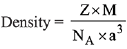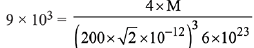M = 305.4 x 10-4 = 0.0305 kg/mol

Q.51. The radius of the largest sphere which fits properly at the centre of the edge of a body centred cubic unit cell is:
(Edge length is represented by ‘a’)    (2019)
(a) 0.027 a
(b) 0.047 a
(c) 0.134 a
(d) 0.067 a

Ans. d
For BCC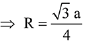∴ Empty space at edge = a - 2R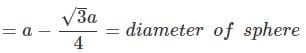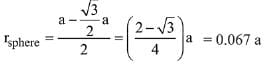Q.52. Element 'B' forms ccp structure and 'A' occupies half of the octahedral voids, while oxygen atoms occupy all the tetrahedral voids. The structure of bimetallic oxide is:    (2019)
(a) A2BO4
(b) AB2O4
(c) A2B2O
(d) A4B2O

Ans. b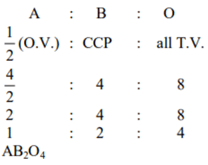Q.53. Consider the bcc unit cells of the solids 1 and 2 with the position of atoms are shown below. The radius of atom B is twice that of atom A. The unit cell edge length is 50% more in solid 2 than in 1. What is the approximate packing efficiency in solid 2?    (2019)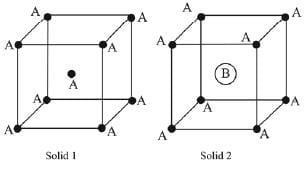(a) 45%
(b) 75%
(c) 90%
(d) 65%

Ans. c
Volume occupied by atoms in solid 2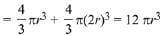Relationship between body diagonal and radius of atom (r),
6r = √3 a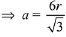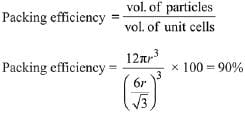Q.54. An element has a face-centred cubic (fcc) structure with a cell edge of a. The distance between the centres of two nearest tetrahedral voids in the lattice is:    (2019)
(a) √2a
(b) a
(c) a/2
(d) 3/2 a

Ans. c
In FCC, tetrahedral voids are located on the body diagonal at a distance of √3a/4 from the corner. Together they form a smaller cube of edge length a/2. Therefore, distance between centres of two nearest tetrahedral voids in the lattice is also a/2.

Q.55. The ratio of number of atoms present in a simple cubic, body centered cubic and face centered cubic structure are, respectively:    (2019)
(a) 8 : 1 : 6
(b) 1 : 2 : 4
(c) 4 : 2 : 1
(d) 4 : 2 : 3

Ans. b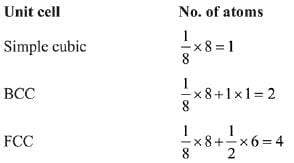Q.56. 10 mL of 1 mM surfactant solution forms a monolayer covering 0.24 cm2 on a polar substrate. If the polar head is approximated as a cube, what is its edge length?    (2019)
(a) 1.0 pm
(b) 2.0 pm
(c) 0.1 nm
(d) 2.0 nm

Ans. b
No. of surfactant molecules = 6 × 1023 × 10/1000 × 10-3 = 6 × 1018 molecule
Let edge length = a cm
Total surface area of surfactant = 6 × 1018 a2 = 0.24 cm2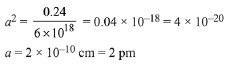Q.57. Which type of ‘defect’ has the presence of cations in the interstitial sites?    (2018)
(a) Schottky defect
(b) Vacancy defect
(c) Frenkel defect
(d) Metal deficiency defect

Ans. c
In Frenkel defect, smaller ion displaces from its actual lattice site into the interstitial sites.

Q.58. Which of the following arrangements shows the schematic alignment of magnetic moments of antiferromagnetic substance?    (2018)
(a)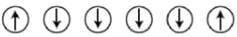(b)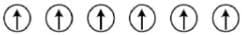(c)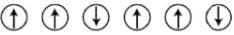(d)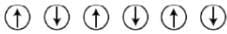Ans. d
Basic knowledge of Antiferromagnetic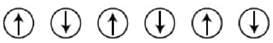Q.59. A metal crystallises in a face centred cubic structure. If the edge length of its unit cell is 'a', the closest approach between two atoms in metallic crystal will be    (2017)
(a) 2a
(b) 2√2a
(c) √2a
(d) a/√2

Ans. d
In FCC, one of the face is like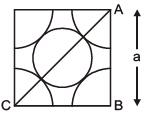By ΔABC,
2a2 = 16r2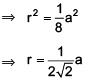Distance of closest approach = 2r = a/√2

Q.60. Which of the following compounds is metallic and ferromagnetic?    (2016)
(a) TiO2
(b) CrO2
(c) VO2
(d) MnO2

Ans. b
CrO2 is metallic and ferromagnetic in nature.

The document JEE Main Previous year questions (2016-22): The Solid State & Surface Chemistry - Notes | Study Chemistry for JEE - JEE is a part of the JEE Course Chemistry for JEE.
All you need of JEE at this link: JEE

## Chemistry for JEE

322 videos|586 docs|368 tests
 Use Code STAYHOME200 and get INR 200 additional OFF

## Chemistry for JEE

322 videos|586 docs|368 tests

Track your progress, build streaks, highlight & save important lessons and more!

,

,

,

,

,

,

,

,

,

,

,

,

,

,

,

,

,

,

,

,

,

;